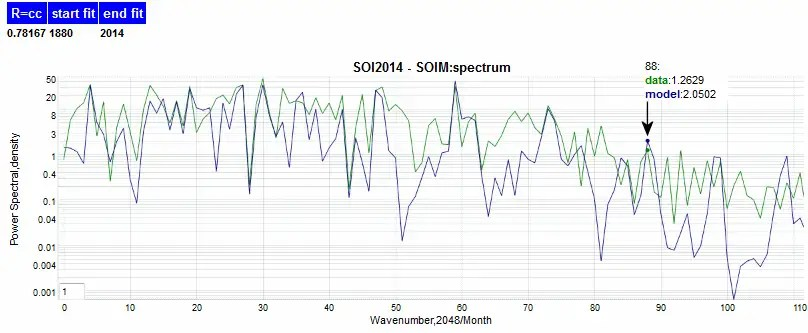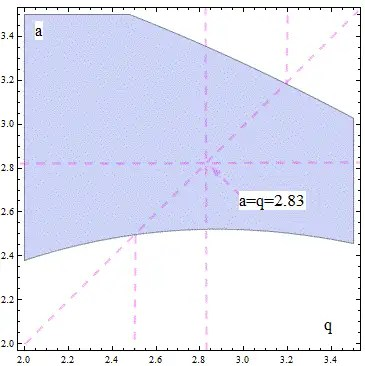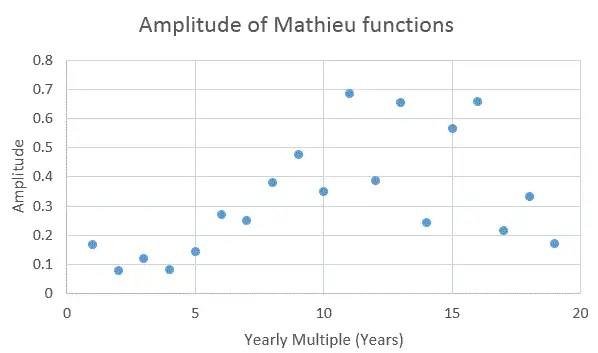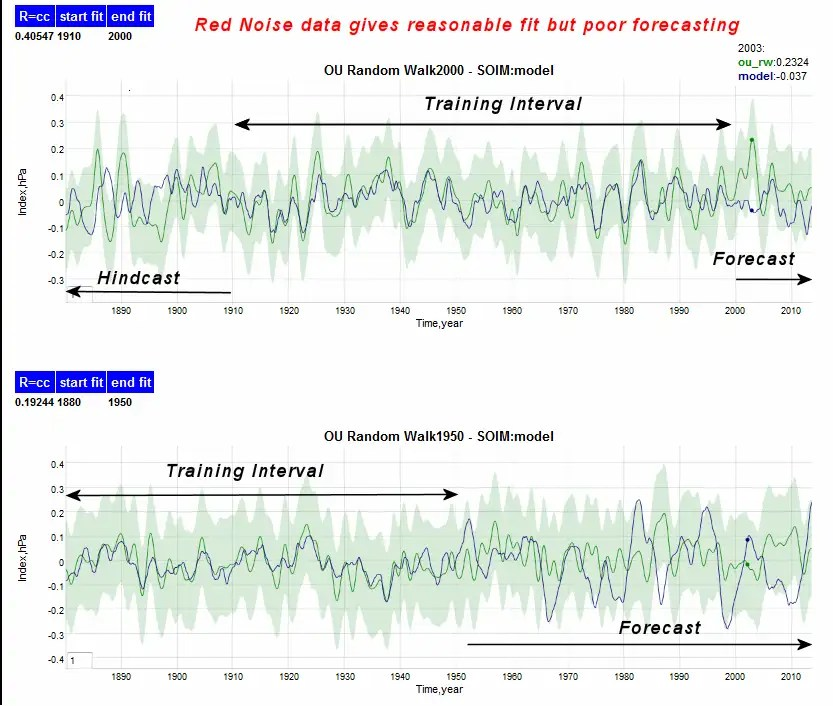# SOIM and the Paul Trap

[mathjax]This post follows up on the idea of modeling the historical Southern Oscillation Index (SOI) record with details on how one can apply the SOIM to make accurate predictions.  Based on some some early encouraging success, I asserted that a more comprehensive model fitting would be possible.  That’s what this follow-on post is about — trying to verify that we can accomplish that “holy-grail” of prediction, the prediction of future El Nino / Southern Oscillation (ENSO) conditions.

To foreshadow what’s to come, Figure 1 shows the comprehensive SOIM fit, which incorporates a grouping of optimally phased Mathieu functions (introduced in the previous post)Fig. 1 : Fit of the full SOI historical record (in green) to the SOI Model (in blue).

This is a very promising result based on the premise of the last post. The principal additions to the simple model are (1) a multi-harmonic basis set of Mathieu functions and (2) a more constraining physical interpretation to the math.

What follows is the explanation and various verification checks, which include:

1. Sensitivity of the model to parameter selection
2. Comparison to fitting red noise (to show over-fitting is not an issue)
3. Hindcasts and forecasts based on restricted training intervals
4. Power spectrum of model and data

Previously, the SOI was modeled as a periodic formulation of the Mathieu function with a fundamental frequency T of approximately 6.3 years.    In practice, the strong fundamental forcing frequencies should appear as harmonics of 1 year intervals, i.e. 1,  2, 3, 4, 5, 6, etc years, based on the yearly seasonal cycle, reinforced by solar and tidal variations.  We first reproduce the equation from the last post, modified to include harmonics of the fundamental.

$$frac{d^2Phi}{deta_n^2} = – [a-2qcos(2eta_n)]Phi$$

This is the essential Mathieu equation, with the periodic element

$$eta_n = frac{n 2 pi t}{T}$$

where T is the yearly fundamental. Even though these are multiples of a yearly interval, they do not emerge as oscillations that are observed on the same cyclic intervals. Instead, due to the non-linear elliptical ODE that the Mathieu functions solve for, the periods are closer to a fundamental angular frequency approximated by the following (in the limit as q goes to zero   ) :

$$omega = sqrt{a}$$

In addition to this approximate fundamental frequency, there exist rich harmonics and overtones (departing from multiples of the harmonic) which produce an inharmonic waveform similar to the following:Fig. 2 : Oscilloscope trace of an inharmonic waveform produced by a short 25 millisecond audio recording of a cymbal. This is not noise, but the equivalent of harmonic waveforms generated by a generalized elliptical surface.

The result is that the fundamental radial frequency will produce peaks and valleys  — not on yearly boundaries — but on multiple intervals related to the a characteristic factor or eigenvalue.   From the previous post, we found :

$$a = 2.83$$

and so the approximate series harmonics are

$$T_4 = frac{4}{sqrt{2.83}} = 2.38 years$$

$$T_5 = frac{5}{sqrt{2.83}} = 2.97 years$$

$$T_6 = frac{6}{sqrt{2.83}} = 3.57 years$$

This means that a first-order reconstructed waveform would be a Fourier series of

$$f(t) = .. + cos({ frac{2 pi}{T_4} t + phi_4}) + cos({ frac{2 pi}{T_5} t + phi_5}) + cos({ frac{2 pi}{T_6} t + phi_6}) + ..$$

These harmonics in fact appear in the power spectrum of the SOI time series, shown below as Figure 3, along with enough extra spectral lines to give the impression of red noise.Fig 3: Power spectrum of the SOI time series. The spectrum is quite rich, indicative of red noise, but closer inspection shows that many peaks are harmonically related.

The next question is how do we arrive at a value of a = 2.83 ? And why does q approximately equal a?

Recall from the last post, that the elliptical ODE given by the first equation corresponds to the wave equation, which in turn represents a classical Hamiltonian or quantum mechanical view of the conservation of energy within the system (see Baez’s Azimuth blog for further insight). In basic terms, the second derivative with respect to time represents the kinetic energy and the linear factor represents the potential — in this case gravitational — energy. Arfken describes a quantum pendulum example in , where in the case of a = q, the average energy is half the potential energy of the system.  This is often referred to as the Virial theorem and is really a thermodynamic or statistical mechanical result related to the system fluctuating about a mean free energy.

This  solves one piece of the puzzle. But why does a = 2.83?

To answer that, we have to find the stable, real values of the Mathieu equation. Not all values of a and q are allowable, and much like the energy bandgap which exists in electronic structure mentioned in the previous post, the real-values of a and q exist in an equivalent band structure, see Figure 4 below:Fig 4: Only certain values of a and q are allowable according to real value solutions to the Mathieu equation. These exist in the blue shaded “band-gap” regions. The diagonal value indicates a=q, where in a classical oscillator, the mean kinetic energy is half the potential energy. Stable values exist where the diagonal and blue regions intersect.

The allowable values for a and q exist within the blue shaded regions and are further constrained by the partitioning of the energy as half the potential energy, see the line lableled “mean equipotential energy line” in Figure 4. The lower energy bound is estimated at a=q=2.496 and the higher energy bound is a=q=3.187.  The median point between the bounds is (2.496+3.187)/2 = 2.8415 which is very close to the empirical value estimated from fitting the SOI data to the Mathieu equation:Fig 5 : Blow up allowable regions of a and q. The value of 2.83 occupies a central sweet spot between the lower and upper energy boundaries.

This analysis is related to a practical example derived from a mass spectroscopy application, something I am familiar with from dissertation work. In a “Paul trap” mass spectroscopy instrument, the ionized material is held aloft in a  radio-frequency band electric field specially configured as a 2D quadropole. The geometry of the field creates hyperbolic contours while the time varying energy imparted to the ion allows it to occupy a stable position in the a vs q state space. Practically speaking, the meta-stable positioning of the ion allows higher sensitivity in the resolution if the mass spectrometer when discriminating between different elements.

The importance of this example is illustrated by the mechanical analog of the rf Paul trap, see Figure 6 below. Here a ball can be forced into a complex orbit by rotating the reference frame at an appropriate rate.Figure 6: The mechanical analog of the rf Paul Trap is a hyperbolic saddle surface with a ball placed on the metastable point. As the reference frame rotates at the proper rate, the ball will stay on the surface, but follow a complicated elliptical route.

We can test some of these concepts out with a few Physics 101 first-order calculations.  In the open ocean, the tides have an amplitude of approximately half meter height (and under El Nino conditions the mean sea level can temporarily increase by 0.1-0.3 m. )  Assuming independent particles spinning at the earth’s radius of 6400 kilometers and yearly frequency, the average rotational kinetic energy is 5 joules per kilogram .  The potential energy of a particle held aloft at 0.5 meter assuming a gravitational constant of 9.8 m/s^2 is 4.9 joules per kilogram. So the exchange of energy between rotational kinetic energy and potential energy implicit in the Mathieu equation exists.

For the Paul trap equations of motion shown below, replace an electric field with a gravitational field and one can see the first-order equivalence and that a=q is feasible and actually necessary to generate a stable orbit or trap.

$$ddot{eta}+(a-2qcos(2tau))eta, a=frac{4eU}{mr^2_0 omega^2}, q=frac{-2eV}{mr^2_0omega^2}, tau=frac{omega t}{2}$$

Where U = 0.5 V , a = q, the solution region is shown below in Figure 7.  This is the practical application of the Paul trap physics proposed for the SOI, replacing charged particles in a rotating elliptical field, with particles under the force of gravity on a spinning earth with an elliptical coordinate system.Fig 7 : For the Paul trap, the forcing function fields interact with the solution of the Mathieu equation to create intersecting solution regions. For this case, one can see the intersection near a=q=2.8 as well as near the origin.

The next step is to actually put the Mathieu functions to practice and see how far we can extend a model fit.  The general idea is to combine a set of basis Mathieu functions, corresponding to sine and cosine functions.  The combination of these functions is nowhere near as intuitive as a Fourier series of sine and cosine pairs, as the parametric plot in Figure 8 shows.Fig 8 : For a cosine vs sine parametric plot, the trajectory traces out a perfect circle. However, for the even/odd MathiueC vs MathieuS parametric plot, the trajectory cycles in odd patterns depending on the choice of a and q. This particular plot is for a=q=2.82.

Interestingly, this idea is similar to that proposed in 1929 by Goldstein in a paper called “Rotating Elliptic Basins of Constant Depth”   but he didn’t pursue it much further due to the difficulty in tabulating Mathieu functions without the computational support we have gained in the 80 subsequent years.  Antenucci and Imberger  have at least read Goldstein’s work and have applied the ideas to large lakes.   Before I get to that detail in a later post, let’s see how we can improve in the first-order view.

## Full Analysis and Results

The first thing to clear up is that since the full elliptical solution is a spatio-temporal PDE, the fact that the Darwin and Tahiti pressure readings can be out of phase by 180 degrees is perfectly acceptable; this means that the SOI, which is the difference between the Tahiti and Darwin readings, works as effectively and perhaps better than the Darwin reading alone. As the previous post applied Darwin data, we can apply the difference without loss o generality.

The remaining unknown factor in fitting a set Mathieu functions to the SOI time series is in determining which yearly multiple provides sufficient forcing to generate a parametric resonance in the output. Since we don’t know whether it is the 1 year multiple (the obvious case) or some other multiple such as 7 years (the period often observed in spectral analysis of SOI data), we take the approach of fitting to a full set and letting a solver find the best combination.  Figure 9 below shows the set of amplitudes used to fit Figure 1 generated from the same algorithm used in the CSALT model.Figure 9: The set of Mathieu function amplitudes (phases not indicated) used to generate a fit to the SOI time series.

This is truncated at 19 years assuming that additional multiples will start to have diminishing returns. The correlation coefficient of 0.77 is good but that could also be the result of over-fitting.    One way to check this is based on using training intervals to validate regions not influenced by the training, starting with Figure 10.Fig 10 : SOI data used as a training interval for both forecasting and hindcasting. This works robustly even though only half the data is used in either direction. Predicted peak position is more accurate than predicted peak amplitude.

When the training period is extended, the fit becomes more impressive in Figure 11:Fig 11: Larger training interval improves the model fit.

And Figure 12 is a combined forecast and hindcast based on a middle training intervalFig 12 : Middle region training interval

To check that over-fitting is not an issue, we applied the algorithm to artifiically generated red noise data in Figure 13.Fig 13 : Fitting Mathieu functions to red noise data gave reasonable fits in the training interval but lost coherence in both forecasts and hindcasts, suggesting that the SOI is better modeled as a deterministic (i.e. non random) phenomenon.

And if instead of using the Mathieu functions for the basis set, we apply pairs of sines and cosines of the same characteristic parameter, we get the terrible model fits of Figure 14.Fig 14 : Instead of Mathieu functions, a set of sin + cosine Fourier series is used for the training interval, the forecasts become useless.

This phenomena of forecasts blowing up is very common when sine waves of slightly different frequencies (i.e. 10, 11, 12 year periods) are applied during the fit — as any phase optimization that is used during the fit becomes counterproductive as the waveforms eventually get out of phase.  This is not a problem with Mathieu functions as slightly different characteristic frequencies have substantially different overtones, see Figure 8, thus preventing phase adjustments from optimizing the fit over the training interval.

The tuning of the SOIM to intervals other than yearly multiples results in fits that are not as optimal, reducing the correlation coefficient from 0.78 to 0.6 with a 5% change in frequency. This is understandable if the underlying frequency is an important aspect of the SOI phenomena.

Finally, we can return to the power spectrum of Figure 3. The model power spectrum matches the data in terms of richness in the peak positions and demonstrates why conventional signal processing techniques fail miserably at recovering the underlying time series behavior.  The residual error shows enough structure in Figure 15 that it is tempting to try to find other elliptical periodicities in the time series.Fig 15 : Residual error in the SOIM used to fit the historical SOI data.

What I still haven’t done is to independently fit Darwin and Tahiti to see if the model is able to extract the spatio-temporal phasing relationships between the two sites. This will be the first step in understanding the full PDE solution.

# Conclusions

The reason that I started looking at predicting the SOI was that the CSALT model for predicting global surface temperatures worked so well, yet it was dependent on the SOI as a thermodynamic variational parameter. And since the SOI was not even close to being predictable, the CSALT model would not be as effective as a prediction tool. But now that the SOI Model — the SOIM — is showing skill at predicting based on training data, it may provide the missing piece to projecting global warming scenarios or sea-level rise  by eliminating the major factor of ENSO natural variations.

Bumper: I took one college course on limnology, had the great Al Nier, the pioneer in mass spectroscopy, teaching me Physics 101, and now this. What an elliptical route this learning path has developed into.

## References

  Arfken, George Brown, Hans-Jurgen Weber, and Lawrence Ruby. Mathematical methods for physicists. Vol. 6. New York: Academic press, 1985. See http://booksite.elsevier.com/9780123846549/index.php for a freely available supplement describing Mathieu function.

 S. Goldstein, “Tidal Motion in Rotating Elliptic Basins of Constant Depth.,” Geophysical Journal International, vol. 2, no. s4, pp. 213–232, 1929.

 J. P. Antenucci and J. Imberger, “Energetics of long internal gravity waves in large lakes,” Limnology and Oceanography, vol. 46, no. 7, pp. 1760–1773, 2001.

 D. A. Jay, “Evolution of tidal amplitudes in the eastern Pacific Ocean,” Geophys. Res. Lett., vol. 36, no. 4, p. L04603, Feb. 2009.

## 9 thoughts on “SOIM and the Paul Trap”

•Keith Pickering

I’m highly dubious of any claimed oscillation in which we have data for less than two complete cycles. Looks like overfitting to me.

Like

1.Keith Pickering

Really fascinating stuff in these last two postings. Some questions:
1. Are you heading for peer-review with this? Because I think you ought to.
2. Do you have R code available, and if so, will it be published here or elsewhere?
3. It would be interesting to see a plot of training interval length vs. correlation of forecast/hindcast. In other words, what we’d really like to know is, how far out can a forecast be “reliable” with a given training interval?
4. The “jackpot” question: what does your forecast show for the next 10 years? Or 20 years?

Like

•admin

Keith,
I would have used R, but the R-library lacks a Mathieu function library. Instead I used Mathematica to generate the time series, and then fed that into the R solver. This is a bit convoluted and so what I will do is at least provide some pseudo-code.

I will do the various training intervals next. This creates a useful variance in outcomes.

This is what I posted at http://realclimate.org for a forecast based on a training interval up to 2000. This shows a strong El Nino peak at the midway point of next year.

Like

•Keith Pickering

Thanks for that. Mathieu functions are part of the latest release of GSL, which is open source, so presumably one could write an R extension rather easily, if one were so inclined. I don’t have Matlab so I have no idea how the passed parms look there, but ideally one would want R to match Matlab’s naming and parm conventions as closely as possible so that the code will port.

I also note in passing that your prediction (at least in the short term) matches the one here: http://havlin.biu.ac.il/PS/Very%20early%20warning%20of%20next%20El%20Nino.pdf
… using a rather different procedure; and also fits this prediction:
http://www.wunderground.com/blog/JeffMasters/an-el-nio-coming-in-2014
… based on yet another completely different analysis.

The difference is, you’re forecasting out *four years*, which puts you way ahead of the pack in my book.

Like

•admin

Keith,
Thanks for those links. R has got a few transcendental functions such as Bessel, so maybe if I do more digging I can find something.

Like

2.admin

Some skeptics are upset with the contents of this post. Like Maksimovich at Climate Etc, who wrote

Inharmonic systems introduce another mechanism synchronization eg

When a string is bowed or tone in a wind instrument initiated by vibrating reed or lips, a phenomenon called mode-locking counteracts the natural inharmonicity of the string or air column and causes the overtones to lock precisely onto integer multiples of the fundamental pitch

Enso can synchronize with the annular mode eg phase locking (Zalipin and Ghil 2010) and is amenable to certain predicates such as Arnold tongues for predicting when predicting is uncertain (Lyapunov (in)stability)

Have you found another mechanism (apart from rediscovering of the wheel)?

I do in fact have something equivalent to an annular mode phase-locking, as all the Mathieu function periods are multiples of a year, aka a parametric resonance.

This is what we call an “Own Goal”. If the point is that I rediscovered the wheel, that is OK, because now there is no excuse to pursue this path to make predictions.

I. Zaliapin and M. Ghil, “Another look at climate sensitivity.,” Nonlinear Processes in Geophysics, vol. 17, no. 2, 2010.

Like